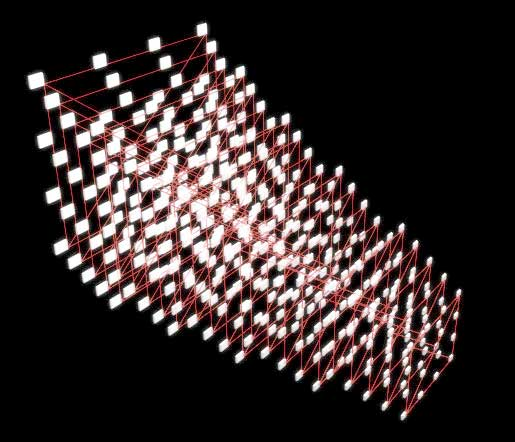Flash projection algorithm

concept of the geometry is describe at
thelayered pixels section

A projection algorithm, for the fragments1 physical setup. The small map on the right shows an agent
moving in 3D, that represents the projection space. The large area consists of 192 boxes on 12 Z layers,
that are showing their 3D distance from the traveling agent with color change and a rotational meter.
1

// Functions
// sense travel, called from main, creates 3D moving agent
function senseTravel() {
____idist = 0;
____for (i=0; i<3; i++) {
_________root.sensePos[i] -= (_root.sensePos[i]-_root.senseTarget[i])/_root.senseSpeed;
________idist += Math.pow((_root.sensePos[i]-_root.senseTarget[i]), 2);
____}
____if (Math.pow(idist, (1/3))<7) {
_________root.senseTarget = [random(limitX), random(limitY), random(limitZ)];
____}
}

// calculateDistance function, returns distance of
// root sense from the x,y, and lightBoxLevel coordinates
// called from every lightBox to determine their color.

function calculateDistance(x, y, z) {
____return Math.pow(Math.pow((x-_root.sensePos), 2)+Math.pow((y-_root.sensePos), 2)+Math.pow((z-_root.sensePos), 2), 0.5);
}

// -----------------the "main method"
_root.onEnterFrame = function() {
____senseTravel();
};A possible spatial layout the above lightBoxes.